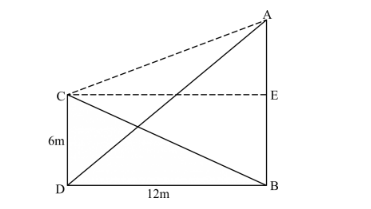# Two poles of height 6 m and 11 m stand vertically upright on a plane ground. If the distance between their foot is 12 m, the distance between their tops is

Question:

Two poles of height 6 m and 11 m stand vertically upright on a plane ground. If the distance between their foot is 12 m, the distance between their tops is

(a) 12 m
(b) 14 m
(c) 13 m
(d) 11 m

Solution:

Given: Two poles of heights 6m and 11m stand vertically upright on a plane ground. Distance between their foot is 12 m.

To find: Distance between their tops.

Let CD be the pole with height 6m.

AB is the pole with height 11m, distance between their foot i.e. DB is 12 m.Let us assume a point E on the pole AB which is 6m from the base of AB.

Hence

AE = AB − 6 = 11 − 6 = 5 m

Now in right triangle AEC, Applying Pythagoras theorem

$\mathrm{AC}^{2}=\mathrm{AE}^{2}+\mathrm{EC}^{2}$

$\mathrm{AC}^{2}=5^{2}+12^{2}$ (since CDEB forms a rectangle and opposite sides of rectangle are equal)

$\mathrm{AC}^{2}=25+144$

$\mathrm{AC}^{2}=169$

$\mathrm{AC}=13 \mathrm{~m}$

Thus, the distance between their tops is 13m.

Hence correct answer is $(c)$.